# NCERT Solutions for Class 9 Maths Chapter 3 Exercise 3.3 Coordinate Geometry

Download NCERT Solutions for Class 9 Maths Chapter 3 Exercise 3.3 Coordinate Geometry. This Exercise contains 2 questions, for which detailed answers have been provided in this note. In case you are looking at studying the remaining Exercise for Class 9 for Maths NCERT solutions for Chapter 4 or other Chapters, you can click the link at the end of this Note.

### NCERT Solutions for Class 9 Maths Chapter 3 Exercise 3.3 Coordinate Geometry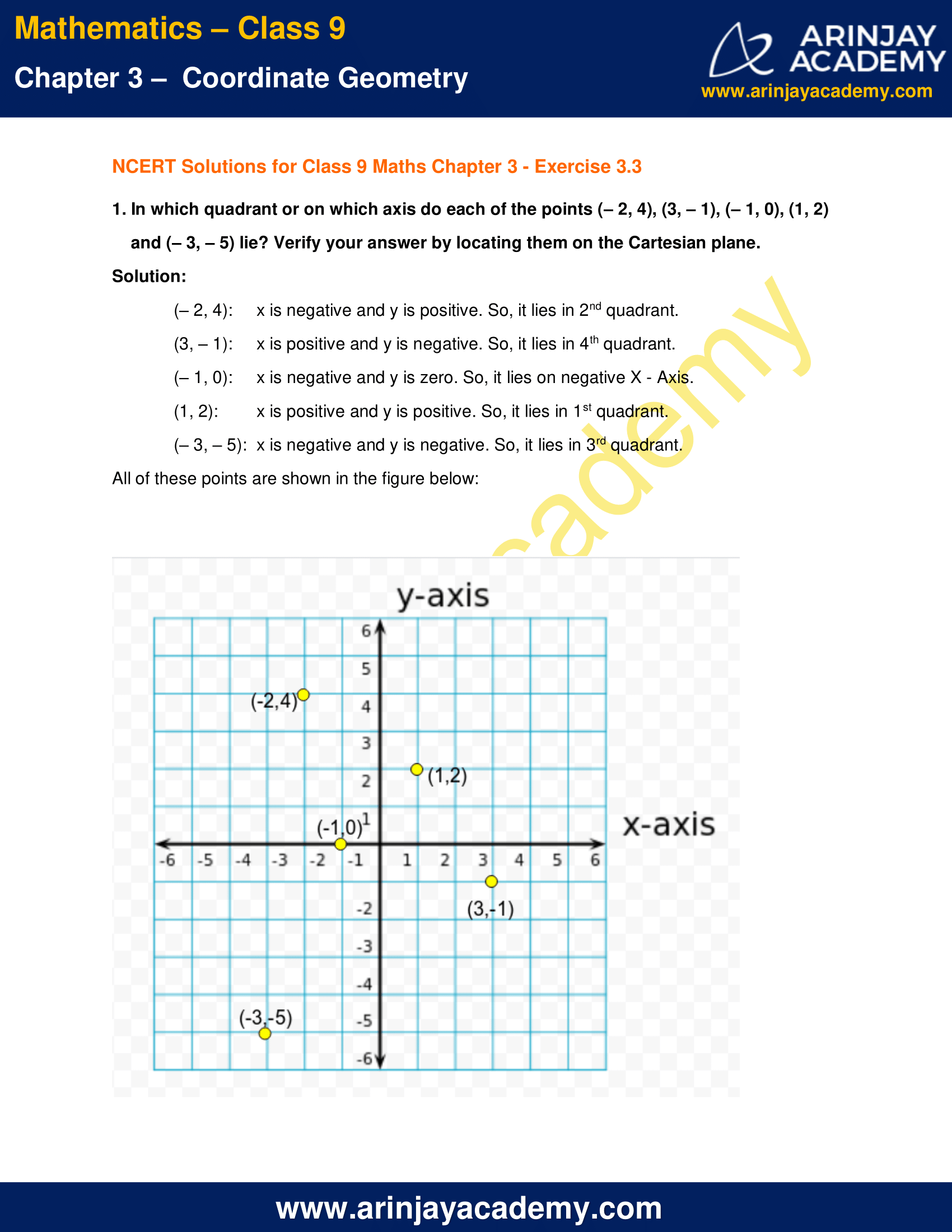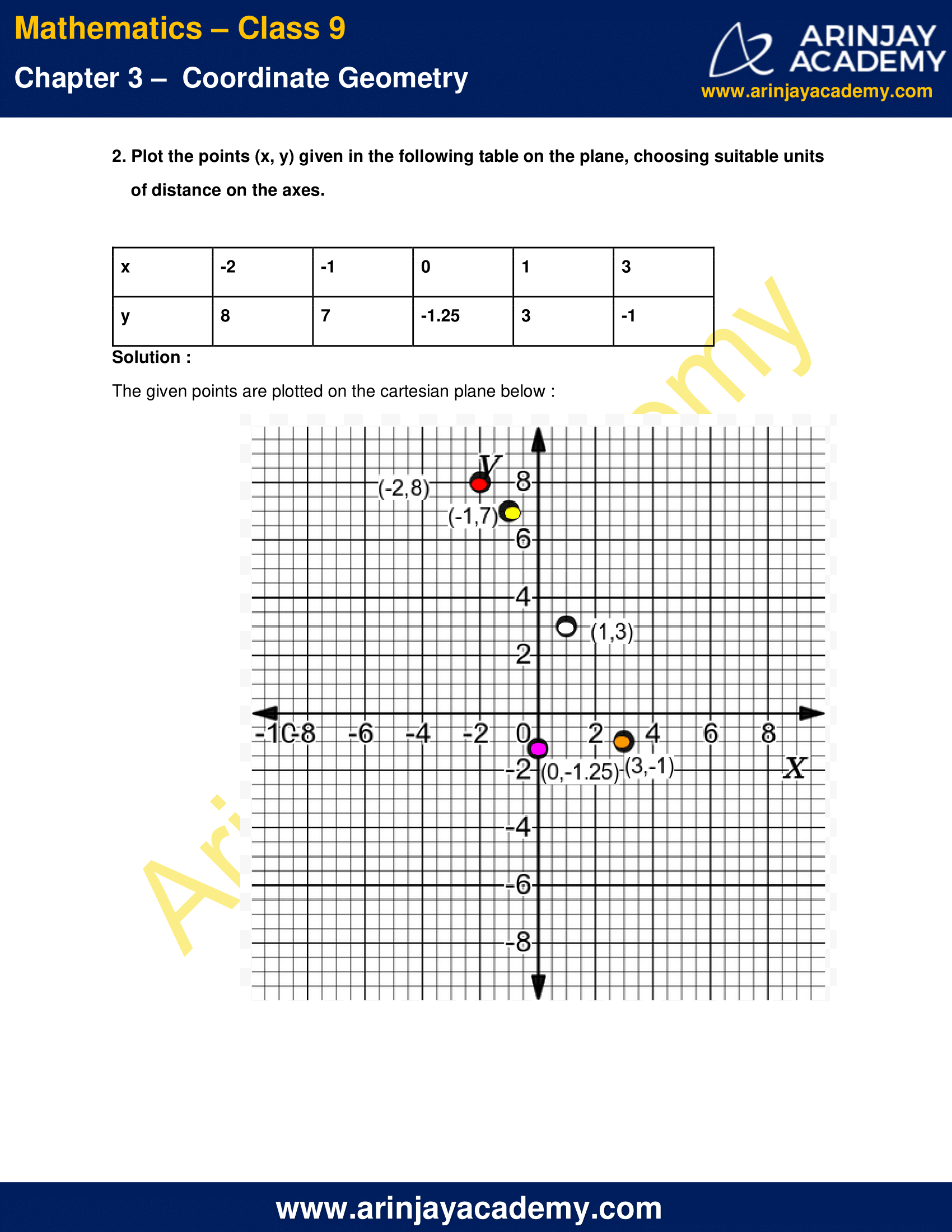NCERT Solutions for Class 9 Maths Chapter 3 Exercise 3.3 Coordinate Geometry

1. In which quadrant or on which axis do each of the points (– 2, 4), (3, – 1), (– 1, 0), (1, 2) and (– 3, – 5) lie? Verify your answer by locating them on the Cartesian plane.

Solution:

(– 2, 4): x is negative and y is positive. So, it lies in 2nd quadrant.
(3, – 1): x is positive and y is negative. So, it lies in 4th quadrant.
(– 1, 0): x is negative and y is zero. So, it lies on negative X – Axis.
(1, 2): x is positive and y is positive. So, it lies in 1st quadrant.
(– 3, – 5):  x is negative and y is negative. So, it lies in 3rd quadrant.
All of these points are shown in the figure below: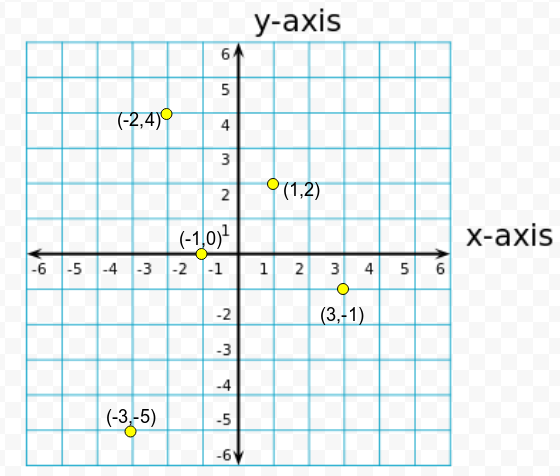2. Plot the points (x, y) given in the following table on the plane, choosing suitable units of distance on the axes.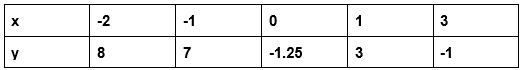Solution :

The given points are plotted on the cartesian plane below :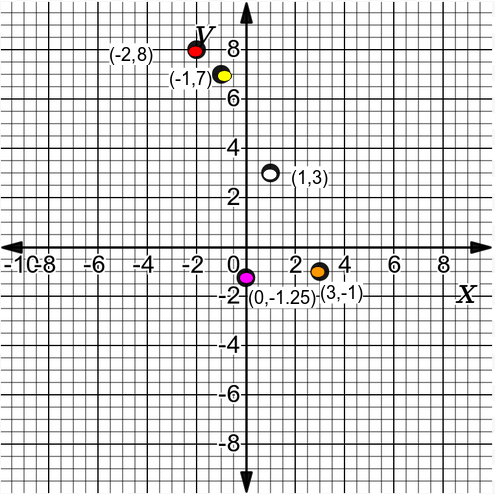NCERT Solutions for Class 9 Maths Chapter 3 Exercise 3.3 – Coordinate Geometry, has been designed by the NCERT to test the knowledge of the student on the topic – Plotting a Point in the Plane if its Coordinates are Given

The next Exercise for NCERT Solutions for Class 9 Maths Chapter 4 Exercise 4.1 – Linear Equations in two Variables can be accessed by clicking here.

Download NCERT Solutions for Class 9 Maths Chapter 3 Exercise 3.3 Coordinate Geometry